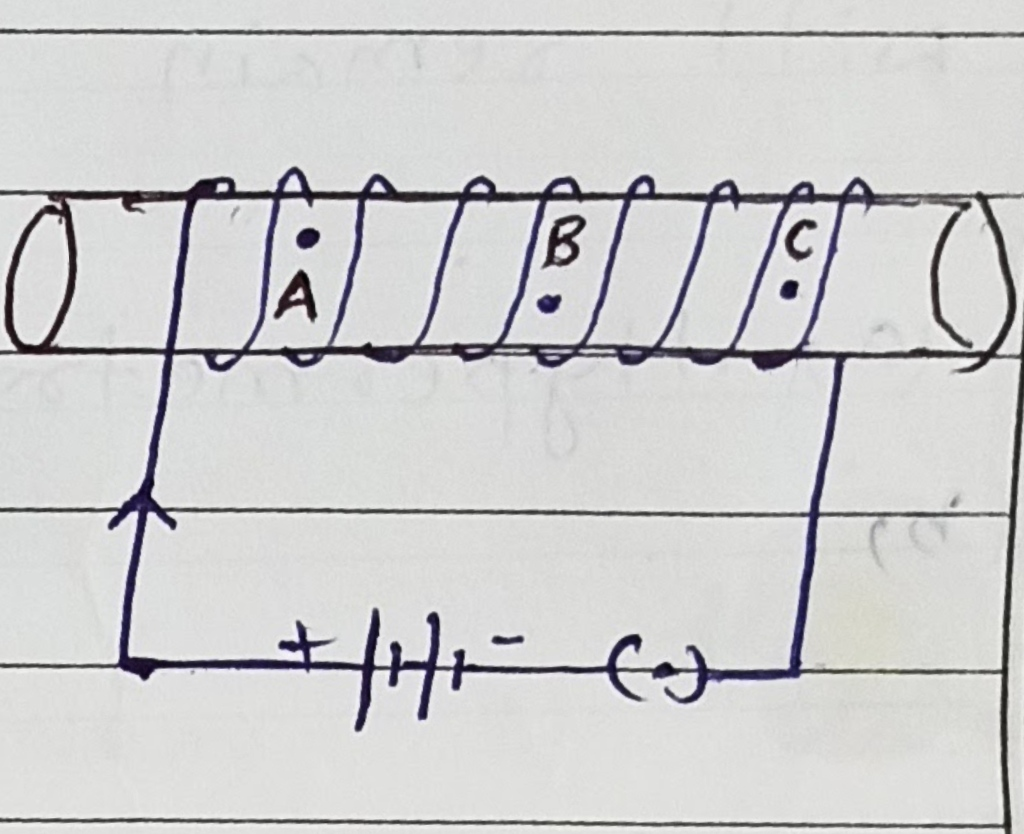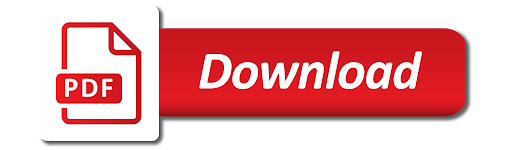# Class 10 Magnetic Effects of Electric Current Important Questions

Magnetic Effects of Electric Current – Important Questions

1. Which of the following represent voltage?
1. Work done/(Current x Time)
2. Work done x Charge
3. (Work done x Time)/Current
4. Work done x Charge x Time
2. Name any two appliances which are based on the application of heating effect of electric current.
3. What is meant by the term frequency of an alternating current? What is the value in India? Why is an alternating current considered to be advantageous over direct current for long range transmission of electric energy?
4. On what factors does the magnitude of magnetic field at a point due to a current carrying circular coil depend?
5. Write the properties of magnetic field lines due to a bar magnet. Why don’t two magnetic field lines intersect each other?
6. .
1. Describe an activity to demonstrate the pattern of magnetic field lines around a straight conductor carrying current.
2. State the rule to find the direction of magnetic field associated with a current carrying conductor?
7. Write the principle of electric generator. How would you convert an AC generator to DC generator?
8. .
1. State Fleming’s left hand rule.
2. Write the principle of working of an electric motor.
3. Explain the function of the following parts of an electric motor.
1. Armature 2. Brushes 3. Split ring
9. Among points A, B and C which are inside a solenoid, which point will have maximum strength of magnetic field? Why?